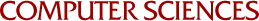## Algorithms for Partial Fraction Decomposition and Rational Function Integration

Ellis Horowitz
1970

Algorithms for symbolic partial fraction decomposition and indefinite integration of rational functions are described. Two types of partial fraction decomposition are investigated, square-free and complete square-free partial fraction decomposition. A method is derived, based on the solution of a linear system, which produces the square-free decomposition of any rational function, say A/B. The computing time is shown to be O(n (In nf) ) where deg(A) < deg(B) = n and f is a number which is closely related to the size of the coefficients which occur in A and B. The complete square-free partial fraction decomposition can then be directly obtained and it is shown that the computing time for this process is also bounded by O(n (In nf)). A thorough analysis is then made of the classical method for rational function integration, due to Hermite. It is shown that the most efficient implementation of this method has a computing time of O(k n (In nc) ), where c is a number closely related to f and k is the number of square-free factors of B. A new method is then presented which avoids entirely the use of partial fraction decomposition and instead relies on the solution of an easily obtainable linear system. Theoretical ana1ysis shows that the computing time for this method is O(n (In nf) ) and extensive testing substantiates its superiority over Hermite's method.